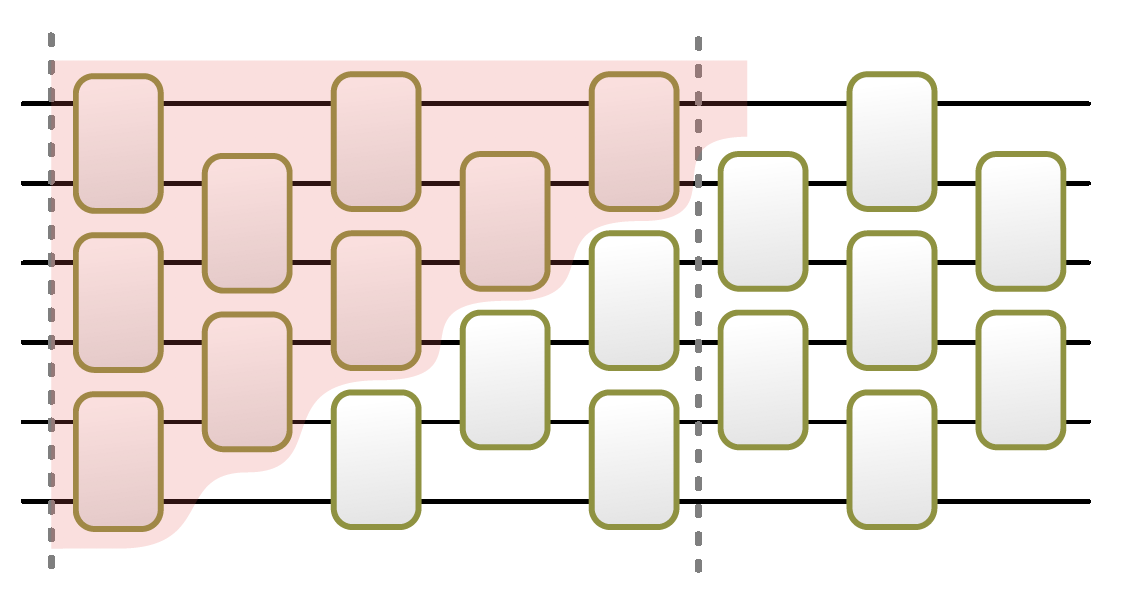Springe direkt zu Inhalt

# Proof of the Brown Susskind conjecture in Nature PhysicsThe Brown Susskind conjecture for random circuits postulates a linear growth of the circuit complexity, until a time exponential in the system size.

Quantifying quantum states' complexity is a key problem in various subfields of science, from quantum computing to black-hole physics. In our work in Nature Physics we prove a prominent conjecture by Brown and Susskind about how random quantum circuits' complexity increases. Consider constructing a unitary from Haar-random two-qubit quantum gates. Implementing the unitary exactly requires a circuit of some minimal number of gates - the unitary's exact circuit complexity. We prove that this complexity grows linearly in the number of random gates, with unit probability, until saturating after exponentially many random gates. Our proof is surprisingly short, given the established difficulty of lower-bounding the exact circuit complexity. Our strategy combines differential topology and elementary algebraic geometry with an inductive construction of Clifford circuits.

This work has received substantial attention and it has been greeted by several articles in the popular press and by press releases. This work is also the first in the group to hit over 100 scites on Scirate.

News from Apr 02, 2022

15 / 100